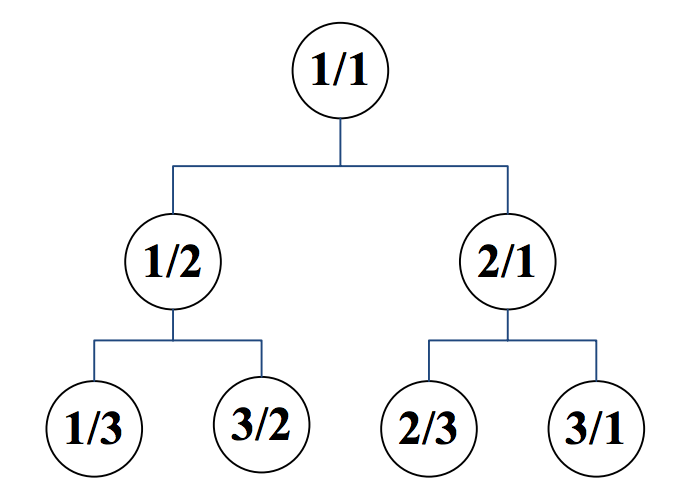# Sequence

Time Limit: 1 Second    Memory Limit: 65536 KB

We inductively label the nodes of a rooted binary tree with an infinite number of nodes as follows:

• The root is labeled by 1/1,
• If the label of a node is p/q, then
• The label of its left child is p/(p+q), and
• The label of its right child is (p+q)/q.
•By having this tree in our hand, we define a rational sequence a1, a2, a3, … by a breadth first traversal of the tree in such a way that nodes in the same level are visited from left to right. Therefore, we have a1=1/1, a2=1/2, a3=2/1, a4=1/3, a5=3/2, …

You are to write a program that gets values p and q and computes an integer n for which an=p/q.

## Input

The first line of the input includes the number of test cases, 1≤t≤1000. Each test case consists of one line. This line contains p, followed by / and then q without any space between them.

## Output

For each test case, output in one line an integer n for which an=p/q. It is guaranteed that in all test cases n fits in a 32-bit integer.

## Sample Input

```4
1/1
1/3
5/2
2178309/1346269
```

## Sample Output

```1
4
11
1431655765
```
Submit

Source: 13th Iran Nationwide Internet Contest - Final